[This article was first published on R – Daniel Oehm | Gradient Descending, and kindly contributed to R-bloggers]. (You can report issue about the content on this page here)
Want to share your content on R-bloggers? click here if you have a blog, or here if you don't.There are a few ways to reduce the dimensions of large data sets to ensure computational efficiency such as backwards selection, removing variables exhibiting high correlation, high number of missing values but by far the most popular is principal components analysis. A relatively new method of dimensionality reduction is the autoencoder. Autoencoders are a branch of neural network which attempt to compress the information of the input variables into a reduced dimensional space and then recreate the input data set. Typically the autoencoder is trained over number of iterations using gradient descent, minimising the mean squared error. The key component is the “bottleneck” hidden layer. This is where the information from the input has been compressed. By extracting this layer from the model, each node can now be treated as a variable in the same way each chosen principal component is used as a variable in following models.

This post will compare the performance of the autoencoder and PCA. For this analysis the Australian Institute of Sport data set will be used which can be found in the DAAG package.

## Principal Components Analysis

PCA reduces the data frame by orthogonally transforming the data into a set of principal components. The first principal component explains the most amount of the variation in the data in a single component, the second component explains the second most amount of the variation, etc. By choosing the top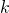principal components that explain say 80-90% of the variation, the other components can be dropped since they do not significantly benefit the model. This procedure retains some of the latent information in the principal components which can help to build better models.

A PCA procedure will be applied to the continuous variables on this data set.

suppressPackageStartupMessages(library(DAAG))
head(ais)

##    rcc wcc   hc   hg ferr   bmi   ssf pcBfat   lbm    ht   wt sex  sport
## 1 3.96 7.5 37.5 12.3   60 20.56 109.1  19.75 63.32 195.9 78.9   f B_Ball
## 2 4.41 8.3 38.2 12.7   68 20.67 102.8  21.30 58.55 189.7 74.4   f B_Ball
## 3 4.14 5.0 36.4 11.6   21 21.86 104.6  19.88 55.36 177.8 69.1   f B_Ball
## 4 4.11 5.3 37.3 12.6   69 21.88 126.4  23.66 57.18 185.0 74.9   f B_Ball
## 5 4.45 6.8 41.5 14.0   29 18.96  80.3  17.64 53.20 184.6 64.6   f B_Ball
## 6 4.10 4.4 37.4 12.5   42 21.04  75.2  15.58 53.77 174.0 63.7   f B_Ball

# standardise
minmax <- function(x) (x - min(x))/(max(x) - min(x))
x_train <- apply(ais[,1:11], 2, minmax)

# PCA
pca <- prcomp(x_train)

# plot cumulative plot
qplot(x = 1:11, y = cumsum(pca$sdev)/sum(pca$sdev), geom = "line")This suggests the first 6 components account for approximately 90% of the variation in the data. The first 2 components will be visualised in a scatter graph. Just for some context male and females will be highlighted.

ggplot(as.data.frame(pca$x), aes(x = PC1, y = PC2, col = ais$sex)) + geom_point()By only visualising 2 principal components were are not seeing all of the variation in the data. Plotting the data points in 3 dimensions gives a better indication of the structure of the data. However, there are still 8 dimensions which explain some of the variation that are not visualised. To do so they would all need to be plotted in their various combinations. This is a draw back of PCA.

pca_plotly <- plot_ly(as.data.frame(pca$x), x = ~PC1, y = ~PC2, z = ~PC3, color = ~ais$sex) %>% add_markers()

This shows how the third dimension separates the males from the females.

## Autoencoder

The same variables will be condensed into 2 and 3 dimensions using an autoencoder. The autoencoder will be constructed using the keras package. As with any neural network there is a lot of flexibility in how autoencoders can be constructed such as the number of hidden layers and the number of nodes in each. With each hidden layer the network will attempt to find new structures in the data. In general autoencoders are symmetric with the middle layer being the bottleneck. The first half of the autoencoder is considered the encoder and the second half is considered the decoder.

# autoencoder in keras
suppressPackageStartupMessages(library(keras))

# set training data
x_train <- as.matrix(x_train)

# set model
model <- keras_model_sequential()
model %>%
layer_dense(units = 6, activation = "tanh", input_shape = ncol(x_train)) %>%
layer_dense(units = 2, activation = "tanh", name = "bottleneck") %>%
layer_dense(units = 6, activation = "tanh") %>%
layer_dense(units = ncol(x_train))

# view model layers
summary(model)

## ___________________________________________________________________________
## Layer (type)                     Output Shape                  Param #
## ===========================================================================
## dense_70 (Dense)                 (None, 6)                     72
## ___________________________________________________________________________
## bottleneck (Dense)               (None, 2)                     14
## ___________________________________________________________________________
## dense_71 (Dense)                 (None, 6)                     18
## ___________________________________________________________________________
## dense_72 (Dense)                 (None, 11)                    77
## ===========================================================================
## Total params: 181
## Trainable params: 181
## Non-trainable params: 0
## ___________________________________________________________________________

# compile model
model %>% compile(
loss = "mean_squared_error",
)

# fit model
model %>% fit(
x = x_train,
y = x_train,
epochs = 2000,
verbose = 0
)

# evaluate the performance of the model
mse.ae2 <- evaluate(model, x_train, x_train)
mse.ae2

##        loss
## 0.009725631

# extract the bottleneck layer
intermediate_layer_model <- keras_model(inputs = model$input, outputs = get_layer(model, "bottleneck")$output)
intermediate_output <- predict(intermediate_layer_model, x_train)

ggplot(data.frame(PC1 = intermediate_output[,1], PC2 = intermediate_output[,2]), aes(x = PC1, y = PC2, col = ais$sex)) + geom_point()The autoencoder is still separating the males from the females in this example however it picks up on structure in the data that PCA does not. Given this is a small example data set with only 11 variables the autoencoder does not pick up on too much more than the PCA. Highly complex data with perhaps thousands of dimensions the autoencoder has a better chance of unpacking the structure and storing it in the hidden nodes by finding hidden features. In contrast to PCA the autoencoder has all the information from the original data compressed in to the reduced layer. To view the data in 3 dimensions the model will need to be fit again with the bottleneck layer with 3 nodes. model3 <- keras_model_sequential() model3 %>% layer_dense(units = 6, activation = "tanh", input_shape = ncol(x_train)) %>% layer_dense(units = 3, activation = "tanh", name = "bottleneck") %>% layer_dense(units = 6, activation = "tanh") %>% layer_dense(units = ncol(x_train)) # summar of model summary(model3) ## ___________________________________________________________________________ ## Layer (type) Output Shape Param # ## =========================================================================== ## dense_73 (Dense) (None, 6) 72 ## ___________________________________________________________________________ ## bottleneck (Dense) (None, 3) 21 ## ___________________________________________________________________________ ## dense_74 (Dense) (None, 6) 24 ## ___________________________________________________________________________ ## dense_75 (Dense) (None, 11) 77 ## =========================================================================== ## Total params: 194 ## Trainable params: 194 ## Non-trainable params: 0 ## ___________________________________________________________________________ # compile model model3 %>% compile( loss = "mean_squared_error", optimizer = "adam" ) # fit model model3 %>% fit( x = x_train, y = x_train, epochs = 2000, verbose = 0 ) # evaluate the model evaluate(model3, x_train, x_train) ## loss ## 0.006243833 # exgtract the bottleneck layer intermediate_layer_model <- keras_model(inputs = model3$input, outputs = get_layer(model3, "bottleneck")$output) intermediate_output <- predict(intermediate_layer_model, x_train) # plot the reduced dat set aedf3 <- data.frame(node1 = intermediate_output[,1], node2 = intermediate_output[,2], node3 = intermediate_output[,3]) ae_plotly <- plot_ly(aedf3, x = ~node1, y = ~node2, z = ~node3, color = ~ais$sex) %>% add_markers()

There is a slight difference between the autoencoder and PCA plots and perhaps the autoencoder does slightly better at differentiating between male and female athletes. Again, with a larger data set this will be more pronounced.

## Comparison of reconstruction error

To compare the the results of PCA and the autoencoder we observe the reconstruction error. This is the mean squared error of the reconstructed input data from it’s reduced state and the true input data. The PCA reconstruction is done by the following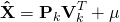where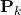is the principal component matrix keeping only the firstprincipal components,is the rotation matrix retaining only the firstcomponents andis the matrix of means.

Reconstruction of the original data by the autoencoder is essentially already done. It is this error which was minimised to construct the reduced set. As mentioned, the first half of the autoencoder is the encoder and the second is the decoder. Since the autoencoder encodes all the information available into the reduced layer, in turn the decoder is better equipped to reconstruct the original data set. The decoder is also a more sophisticated and optimised process than PCA.

The original data set will be reconstructed for the PCA and autoencoder for values.

# pCA reconstruction
pca.recon <- function(pca, x, k){
mu <- matrix(rep(pca$center, nrow(pca$x)), nrow = nrow(pca$x), byrow = T) recon <- pca$x[,1:k] %*% t(pca$rotation[,1:k]) + mu mse <- mean((recon - x)^2) return(list(x = recon, mse = mse)) } xhat <- rep(NA, 10) for(k in 1:10){ xhat[k] <- pca.recon(pca, x_train, k)$mse
}

ae.mse <- rep(NA, 5)
for(k in 1:5){
modelk <- keras_model_sequential()
modelk %>%
layer_dense(units = 6, activation = "tanh", input_shape = ncol(x_train)) %>%
layer_dense(units = k, activation = "tanh", name = "bottleneck") %>%
layer_dense(units = 6, activation = "tanh") %>%
layer_dense(units = ncol(x_train))

modelk %>% compile(
loss = "mean_squared_error",
)

modelk %>% fit(
x = x_train,
y = x_train,
epochs = 5000,
verbose = 0
)

ae.mse[k] <- unname(evaluate(modelk, x_train, x_train))
}

df <- data.frame(k = c(1:10, 1:5), mse = c(xhat, ae.mse), method = c(rep("pca", 10), rep("autoencoder", 5)))
ggplot(df, aes(x = k, y = mse, col = method)) + geom_line()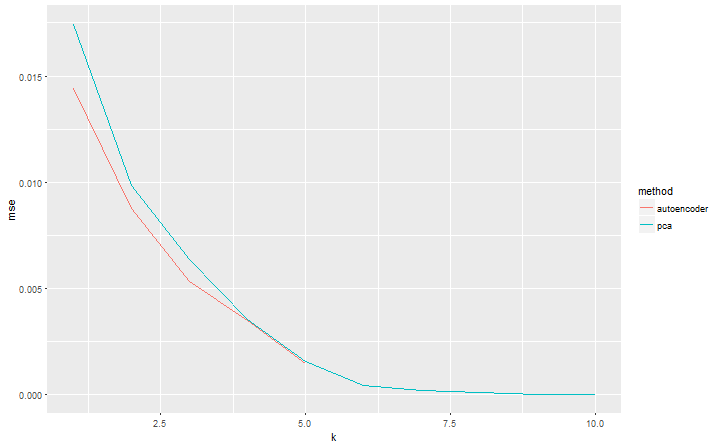What is interesting is the autoencoder is better at reconstructing the original data set than PCA whenis small, however the error converges asincreases. For very large data sets this difference will be larger and means a smaller data set could be used for the same error as PCA. When dealing with big data this is an important property.

## Differences between PCA and autoencoders

To summarise, the key differences for consideration between PCA and autoencoders are:

• There are no guidelines to choose the size of the bottleneck layer in the autoencoder unlike PCA. With PCA, the topcomponents can be chosen to factor in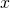of the variation. Often PCA can be used as a guide to choose.
• The autoencoder tends to perform better whenis small when compared to PCA, meaning the same accuracy can be achieved with less components and hence a smaller data set. This is important when dealing with very large data sets.
• When visualising the PCA output, in general the first 2 or 3 components are used. The drawback is the other components are not visable on the plot and therefore not seeing all the information. Different combinations of dimensions will need to be plotted. Autoencoders can be constructed to reduce the full data down to 2 or 3 dimensions retaining all the information which can save time.
• Autoencoders require more computation than PCA. Although, for very large data sets that can’t be stored in memory, PCA will not be able to be performed. The autoencoder construction using keras can easily be batched resolving memory limitations.

## Final thoughts

Autoencoders are my new favorite dimensionality reduction technique, they perform very well and retain all the information of the original data set. They do have draw backs with computation and tuning, but the trade off is higher accuracy. They are great at visualising the data since all the information is retained in 2 or 3 dimensions contrary to PCA. In future posts I’ll look at building an autoencoder for dimensionality reduction from scratch and also look at the maths behind PCA.

The post PCA vs Autoencoders for Dimensionality Reduction appeared first on Daniel Oehm | Gradient Descending.